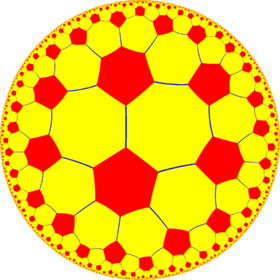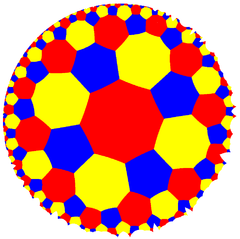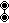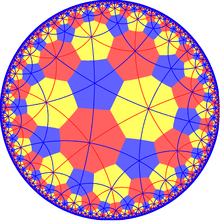# Truncated order-6 square tiling

Truncated order-6 square tilingPoincaré disk model of the hyperbolic plane
TypeHyperbolic uniform tiling
Vertex configuration8.8.6
Schläfli symbolt{4,6}
Wythoff symbol2 6 | 4
Coxeter diagramSymmetry group[6,4], (*642)
[(3,3,4)], (*334)
DualOrder-4 hexakis hexagonal tiling
PropertiesVertex-transitive

In geometry, the truncated order-6 square tiling is a uniform tiling of the hyperbolic plane. It has Schläfli symbol of t{4,6}.

## Uniform coloringsThe half symmetry [1+,6,4] = [(4,4,3)] can be shown with alternating two colors of octagons, with as Coxeter diagram.

## SymmetryTruncated order-6 square tiling with *443 symmetry mirror lines

The dual tiling represents the fundamental domains of the *443 orbifold symmetry. There are two reflective subgroup kaleidoscopic constructed from [(4,4,3)] by removing one or two of three mirrors. In these images fundamental domains are alternately colored black and cyan, and mirrors exist on the boundaries between colors.

A larger subgroup is constructed [(4,4,3*)], index 6, as (3*22) with gyration points removed, becomes (*222222).

The symmetry can be doubled as 642 symmetry by adding a mirror bisecting the fundamental domain.

From a Wythoff construction there are eight hyperbolic uniform tilings that can be based from the regular order-4 hexagonal tiling.

Drawing the tiles colored as red on the original faces, yellow at the original vertices, and blue along the original edges, there are 8 forms.

It can also be generated from the (4 4 3) hyperbolic tilings:Wikimedia Commons has media related to Uniform tiling 6-8-8.